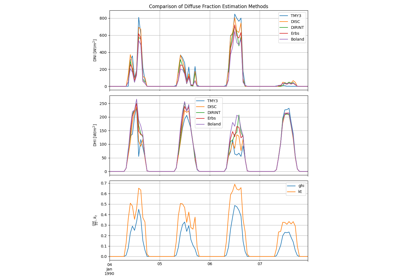pvlib.irradiance.disc(ghi, solar_zenith, datetime_or_doy, pressure=101325, min_cos_zenith=0.065, max_zenith=87, max_airmass=12)[source]#

Estimate Direct Normal Irradiance from Global Horizontal Irradiance using the DISC model.

The DISC algorithm converts global horizontal irradiance to direct normal irradiance through empirical relationships between the global and direct clearness indices.

The pvlib implementation limits the clearness index to 1.

The original report describing the DISC model 1 uses the relative airmass rather than the absolute (pressure-corrected) airmass. However, the NREL implementation of the DISC model 2 uses absolute airmass. PVLib Matlab also uses the absolute airmass. pvlib python defaults to absolute airmass, but the relative airmass can be used by supplying pressure=None.

Parameters
• ghi (numeric) – Global horizontal irradiance in W/m^2.

• solar_zenith (numeric) – True (not refraction-corrected) solar zenith angles in decimal degrees.

• datetime_or_doy (int, float, array, pd.DatetimeIndex) – Day of year or array of days of year e.g. pd.DatetimeIndex.dayofyear, or pd.DatetimeIndex.

• pressure (None or numeric, default 101325) – Site pressure in Pascal. If None, relative airmass is used instead of absolute (pressure-corrected) airmass.

• min_cos_zenith (numeric, default 0.065) – Minimum value of cos(zenith) to allow when calculating global clearness index kt. Equivalent to zenith = 86.273 degrees.

• max_zenith (numeric, default 87) – Maximum value of zenith to allow in DNI calculation. DNI will be set to 0 for times with zenith values greater than max_zenith.

• max_airmass (numeric, default 12) – Maximum value of the airmass to allow in Kn calculation. Default value (12) comes from range over which Kn was fit to airmass in the original paper.

Returns

output (OrderedDict or DataFrame) – Contains the following keys:

• `dni`: The modeled direct normal irradiance in W/m^2 provided by the Direct Insolation Simulation Code (DISC) model.

• `kt`: Ratio of global to extraterrestrial irradiance on a horizontal plane.

• `airmass`: Airmass

References

1

Maxwell, E. L., “A Quasi-Physical Model for Converting Hourly Global Horizontal to Direct Normal Insolation”, Technical Report No. SERI/TR-215-3087, Golden, CO: Solar Energy Research Institute, 1987.

2

Maxwell, E. “DISC Model”, Excel Worksheet. https://www.nrel.gov/grid/solar-resource/disc.html

## Examples using `pvlib.irradiance.disc`#Diffuse Fraction Estimation

Diffuse Fraction Estimation